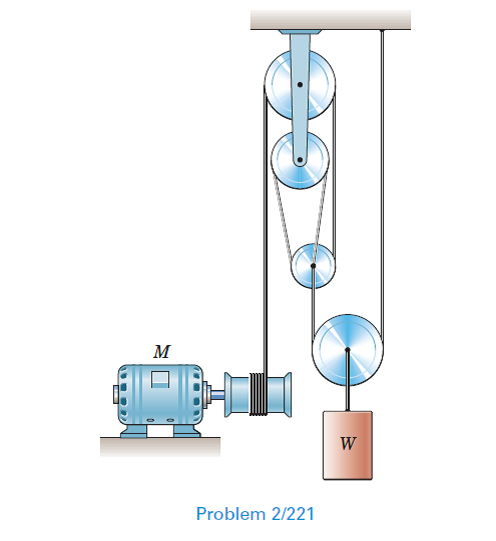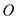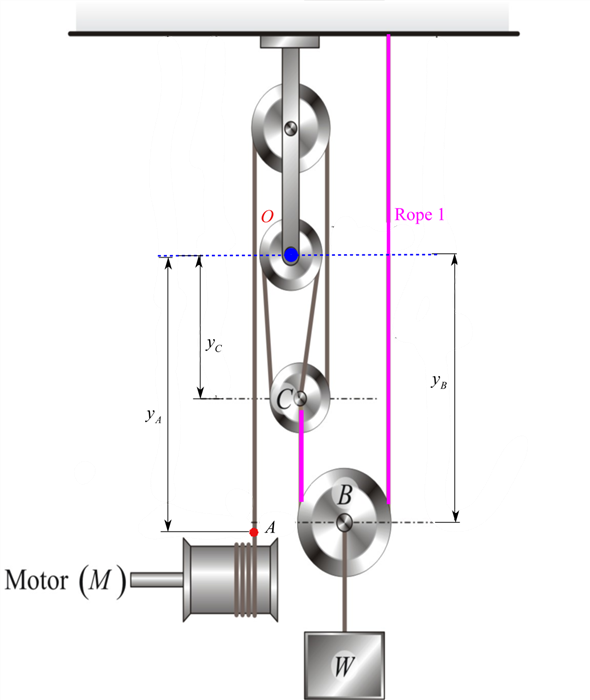Problem

# Determine the vertical rise h of the load W during 10 seconds if the hoisting drum draws...

Determine the vertical rise h of the load W during 10 seconds if the hoisting drum draws in cable at the constant rate of 180 mm /s.#### Step-by-Step Solution

Solution 1

Draw the diagram of the system of the pulleys

Choose an convenient reference pointand the name the distances of the pulleys from the reference pointas shown below.Here, A is any arbitrary point on the rope that is being wrapped on the hoisting drum.

Write the expression for the total length of the rope $$1 .$$

\begin{aligned} L_{1} &=y_{B}+\left(y_{B}-y_{C}\right)+C_{1} \\ &=2 y_{B}-y_{C}+C_{1} \end{aligned}

Here, $$L_{1}$$ is the length of the rope $$1, C_{1}$$ is the constant which refers to the length of the rope wrapped around the circumference of the pulley.

Differentiate the above equation with respect to time.

\begin{aligned} &\frac{d}{d t} L_{1}=\frac{d}{d t}\left(2 y_{B}-y_{C}+C_{1}\right) \\ &0=2 v_{B}-v_{C} \dots (1) \\ &v_{B}=\frac{v_{C}}{2} \end{aligned}

Here, $$v_{C}$$ represents the velocity of the pulley $$C, v_{B}$$ represents the velocity of the pulley $$B$$ (same as the velocity of the load).

Write the expression for the total length of the rope $$2 .$$

$$L_{2}=3 y_{C}+y_{A}+\mathrm{C}_{2}$$

Here, $$L_{2}$$ is the length of the rope $$2, C_{2}$$ is the constant which accounts for the fixed lengths of the cables wrapped around the circumference of the pulleys and the constant vertical separation between the two upper pulleys

Differentiate the above equation with respect to time we have

\begin{aligned} &\frac{d}{d t} L_{2}=\frac{d}{d t}\left(3 y_{C}+y_{A}+C_{2}\right) \\ &0=3 v_{C}+v_{A}+0 \\ &v_{C}=-\frac{v_{A}}{3} \end{aligned}

Here, $$v_{A}$$ represents the velocity of the rope on the hoisting drum.

Substitute $$-\frac{v_{A}}{3}$$ for $$v_{C}$$ in the equation (1).

\begin{aligned} &=-\frac{v_{A}}{3 \times 2} \dots(2) \\ &=-\frac{v_{A}}{6} \end{aligned}

$$v_{B}=\frac{v_{C}}{2}$$

Substitute $$-180 \mathrm{~mm} / \mathrm{s}$$ for $$v_{A}$$ in the equation (2).

\begin{aligned} v_{B} &=-\frac{(-180)}{6} \\ &=30 \mathrm{~mm} / \mathrm{s} \end{aligned}

Therefore, the velocity of the load is $$30 \mathrm{~mm} / \mathrm{s}$$.

Determine the vertical rise of the load using the following relation:

$$h=v_{B} \times t$$

Here, $$t$$ is the time.

\begin{aligned} h &=v_{B} \times t \\ &=30 \times 10 \\ &=300 \mathrm{~mm} \end{aligned}

Therefore, the vertical rise of the load, $$h$$ is $$300 \mathrm{~mm}$$.